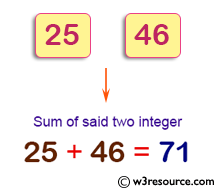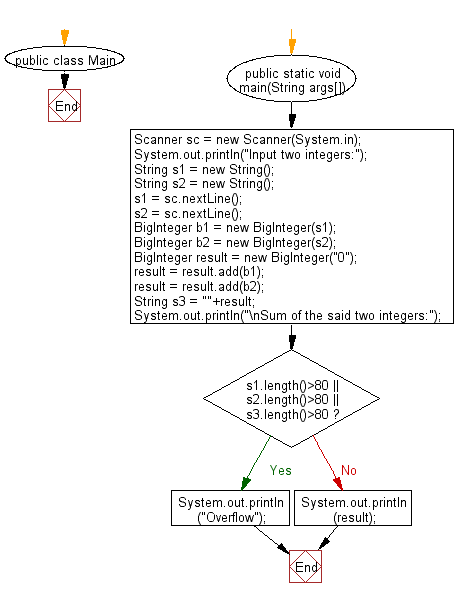# Java Exercises: Compute and print sum of two given integers.

## Java Basic: Exercise-220 with Solution

Write a Java program to compute and print sum of two given integers (more than or equal to zero). If given integers or the sum have more than 80 digits, print "overflow".

Pictorial Presentation:Sample Solution:

Java Code:

`````` import java.*;
import java.math.BigInteger;
import java.util.Scanner;

public class Main {
public static void main(String args[]) {
Scanner sc = new Scanner(System.in);
System.out.println("Input two integers:");
String s1 = new String();
String s2 = new String();
s1 = sc.nextLine();
s2 = sc.nextLine();
BigInteger b1 = new BigInteger(s1);
BigInteger b2 = new BigInteger(s2);
BigInteger result = new BigInteger("0");
String s3 = ""+result;
System.out.println("\nSum of the said two integers:");
if(s1.length()>80 || s2.length()>80 || s3.length()>80)
System.out.println("Overflow");
else
System.out.println(result);
}
}
```
```

Sample Output:

```Input two integers:
25
46

Sum of the said two integers:
71
```

Flowchart:Java Code Editor:

What is the difficulty level of this exercise?

﻿

## Java: Tips of the Day

Array vs ArrayLists:

The main difference between these two is that an Array is of fixed size so once you have created an Array you cannot change it but the ArrayList is not of fixed size. You can create instances of ArrayLists without specifying its size. So if you create such instances of an ArrayList without specifying its size Java will create an instance of an ArrayList of default size.

Once an ArrayList is full it re-sizes itself. In fact, an ArrayList is internally supported by an array. So when an ArrayList is resized it will slow down its performance a bit as the contents of the old Array must be copied to a new Array.

At the same time, it's compulsory to specify the size of an Array directly or indirectly while creating it. And also Arrays can store both primitives and objects while ArrayLists only can store objects.

Ref: https://bit.ly/3o8L2KH